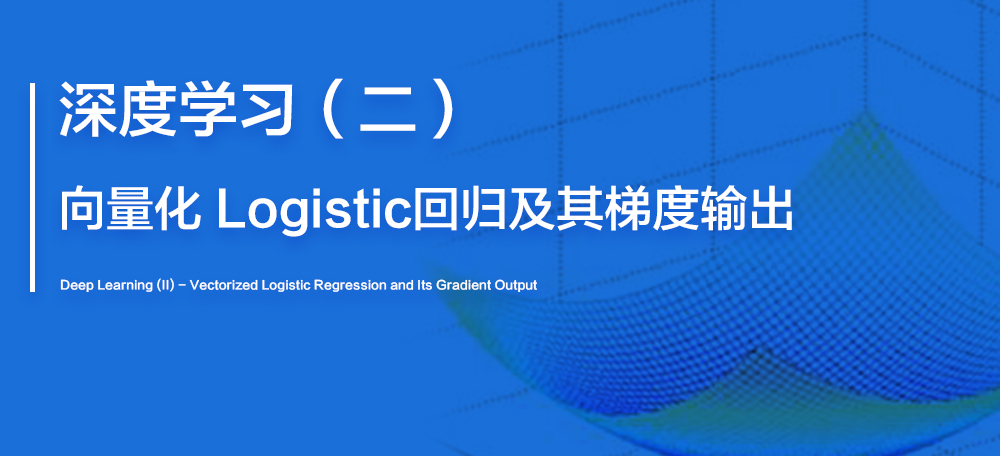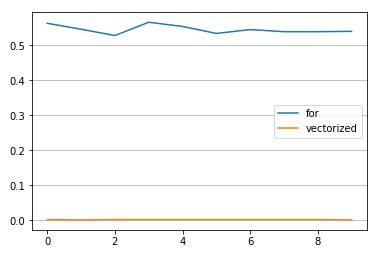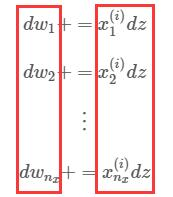# 概述# 2.1 非向量化与向量化实例

## 使用向量化# 2.2 向量化 Logistic 回归

## for 循环版本

1  | $for$ $i$ $=$ $1$ $to$ $m$

2  | $\{$

3  |         $z^{(i)}$ $=$ $w^Tx^{(i)}$ $+$ $b$ # 计算线性输出

4  |         $a^{(i)}$ $=$ $\sigma(z^{(i)})$ # 映射到 $[0,1]$

5  |         $J$ $+=$ $L(a^{(i)},y^{(i)})$ # 记录 Loss Function 的累加

6  |         $dz^{(i)}$ $=$ $a^{(i)}-y^{(i)}$ # 计算 $\frac{\partial L(a,y)}{\partial a} \frac{d\sigma(z)}{dz}$ (前两段”链条”的乘积)

7  |         $for$ $j$ $=$ $1$ $to$ $n_x$

8  |                $dw_j$ $+=$ $x^{(i)}_jdz$ # 累加 $dw$

9  |         $db$ $+=$ $dz$ # 累加 $db$

10 | $\}$

11 | $for$ $j$ $=$ $1$ $to$ $n_x$

12 |         $dw_j$ $=$ $dw_j/m$ # 计算每个输入的 $dw$

13 | $db$ = $db/m$ # 计算 $db$

14 | $J$ = $J/m$ # 计算 $Cost Function$

7  | $for$ $j$ $=$ $1$ $to$ $n_x$

8  |         $dw_j$ $+=$ $x^{(i)}_jdz$ # 累加 $dw$3  | $z^{(i)}$ $=$ $w^Tx^{(i)}$ $+$ $b$

## 向量化版本

1  | $Z$ = $w^TX$ $+$ $b$

2  | $A$ = $\sigma(Z)$

3  | $J$ = $-(1/m)(YlogA$ $+$ $(1-Y)log(1-A))$

4  | $dZ$ = $A$ $-$ $Y$

5  | $dw$ = $(1/m)XdZ^T$

6  | $db$ = $(1/m)dZ^T$

1  | $A$ = $\sigma(w^TX$ $+$ $b)$

2  | $J$ = $-(1/m)(YlogA$ $+$ $(1-Y)log(1-A))$

3  | $dw$ = $(1/m)X(A$ $-$ $Y)^T$

4  | $db$ = $(1/m)(A$ $-$ $Y)^T$

# 2.3 本篇小结

1. 在第一篇中已经建立了 Logistic 回归模型，还有必要做向量化工作吗？（有必要
2. 向量化后的 Logistic 回归能够一次性完成所有样本的一次训练，并完成参数的一次迭代，正确或错误？（正确
3. 下面是向量化版本的 Logistic 回归与梯度输出，其中哪几行实现的是向前传播？哪几行实现的是向后传播？（向前1、2行；向后3、4行
1  | $A$ = $\sigma(w^TX$ $+$ $b)$
2  | $J$ = $-(1/m)(YlogA$ $+$ $(1-Y)log(1-A))$
3  | $dw$ = $(1/m)X(A$ $-$ $Y)^T$
4  | $db$ = $(1/m)(A$ $-$ $Y)^T$
4. 试用向量化技术将下面代码简化成一行，其中 $Fun(a,b) = a + b$，$k_i$ 与 $\mu_i$ 是维度相同的矩阵（$var=(1/n)(k+\mu)$
1  |$for$ $i$ $=$ $1$ $to$ $n$
2  |        $var_i$ $+=$ $Fun(k_i,\mu_i)$
3  |$for$ $i$ $=$ $1$ $to$ $n$
4  |        $var_i$ $=$ $var_i/n$

﻿
0%